# Selina Concise Solutions for Chapter 3 Squares and Square Roots Class 8 ICSE Mathematics

##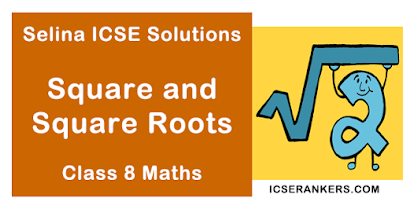### Exercise 3A

1. Find the square of :
(i) 59
(ii) 63
(iii) 15
Solution
(i) Square of 59 = 59 × 59 = 3481
(ii) Square of 6.3 = 6.3 × 6.3 = 39.69
(iii) Square of 15 = 15 × 15 = 225

2. By splitting into prime factors, find the square root of :
(i) 11025
(ii) 396900
(iii) 194481
Solution
(i)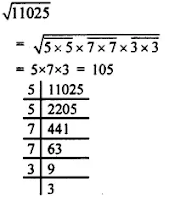(ii)(iii)3. (i) Find the smallest number by which 2592 be multiplied so that the product is a perfect square.
(ii) Find the smallest number by which 12748 be multiplied so that the product is a perfect square?
Solution
(i)On grouping the prime factors of 2592 as shown; on factor i.e., 2 is left which cannot be paired with equal factor.The given number should be multiplied by 2 to make the given number a perfect square.

(ii)On grouping, the prime factors of 12748 as shown; one factor i.e., 3187 is left which cannot be paired with equal factor.The given number should be multiplied by 3187.

4. Find the smallest number by which 10368 be divided so that the result is a perfect square. Also, find the square root of the resulting numbers.
SolutionOn grouping, the prime factors of 10368 as shown; one factor i.e., 2 is left which cannot be paired with equal factor.∴ The given number should be divided by 2.
Now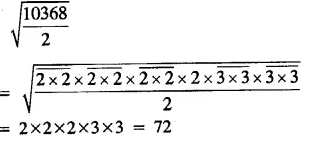5. Find the square root of :
(i) 0.1764
(ii) 96 1/25
(iii) 0.0169
Solution
(i)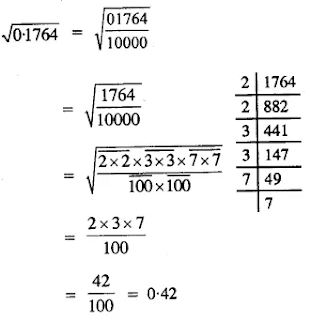(ii)(iii)6. Evaluate:
(i)(ii)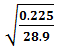(iii)(iv)Solution
(i)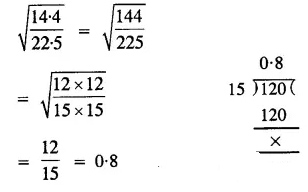(ii)(iii)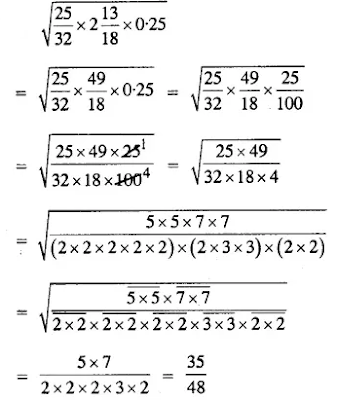(iv)7. Evaluate :
(i)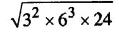(ii)(iii)(iv)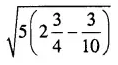(v)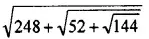Solution
(i)(ii)(iii)(iv)(v)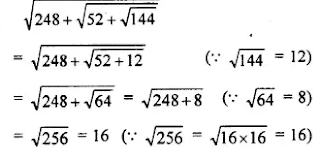8. A man, after a tour, finds that he had spent every day as many rupees as the number of days he had been on tour. How long did his tour last, if he had spent in all  1,296
Solution
Let the number of days he had spent = x
Number of rupees spent in each day = x
Total money spent = x × x = x2 = 1,296 (given)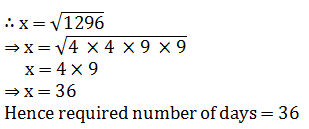9. Out of 745 students, maximum are to be arranged in the school field for a P.T. display, such that the numbers of rows is equal to the number of columns. Find the number of rows if 16 students were left out after the arrangement.
Solution
Total number of students = 745
Students left after standing in arrangement = 16
No. of students who were to be arranged = 745 - 16 = 729
The number of rows = no. of students in each row
No. of rows = √72910. 13 and 31 is a strange pair of numbers such that their squares 169 and 961 are also mirror images of each other. Find two more such pairs.
Solution
(13)2 = 169 and (31)2 = 961
Similarly, two such numbers can be 12 and 21
∴ (12)2 = 144 and (21)2 = 441 and 102, 201
(102)2 = 102 × 102 = 10404
and (201)2 = 201 × 201 = 40401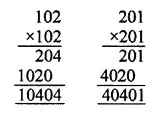11. Find the smallest perfect square divisible by 3, 4, 5 and 6.
Solution
L.C.M.  of 3, 4, 5, 6 = 2 × 2 × 3 × 5 = 60in which 3 and 5 are not in pairs L.C.M. = 2 × 3 × 2 × 5 = 60
we should multiple it by 3 × 5 i.e., by 15
Required perfect square = 60 × 15 = 900

12. If √784 = 28, find the value of:
(i) √7.84 + √78400
(ii) √0.0784 + √0.000784
Solution
(i)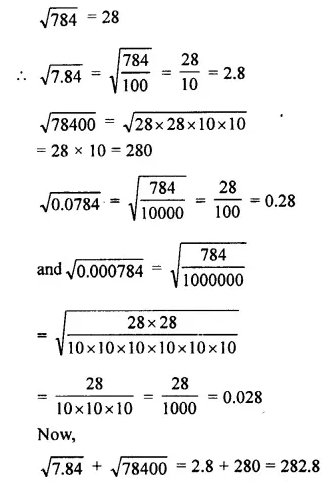(ii)### Exercise 3 B

1. Find the square root of:
(i) 4761
(ii) 7744
(iii) 15129
(iv) 0.2916
(v) 0.001225
(vi) 0.023104
(vii) 27.3529
Solution
(i) 4761Required square root = 69

(ii) 7744Required square root = 88

(iii) 15129Required square root = 123

(iv) 0.2916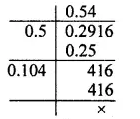Required square root = 0.54

(v) 0.001225Required square root = 0.035

(vi) 0.023104Required square root of 0.152

(vii) 27.3529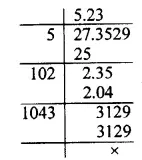Required square root = 5.23

2. Find the square root of:
(i) 4.2025
(ii) 531.7636
(iii) 0.007225
Solution
(i) 4.2025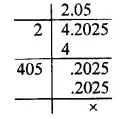Required square root = 2.05

(ii) 531.7636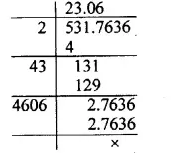Required square root = 23.06

(iii) 0.007225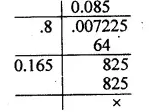Required square root = 0.085

3. Find the square root of:
(i) 245 correct to two places of decimal.
(ii) 496 correct to three places of decimal.
(iii) 82.6 correct to two places of decimal.
(iv) 0.065 correct to three places of decimal.
(v) 5.2005 correct to two places of decimal.
(vi) 0.602 correct to two places of decimal
Solution
(i) 245Required square root = 15.65 upto two places of decimal.

(ii) 496Required square root = 22.2708 = 22.271 upto three places of decimal.

(iii) 82.6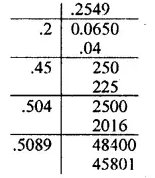Required square root = 9.088 = 9.09 upto two places of decimal.

(iv) 0.065Required square root = .255 upto three places of decimal.

(v) 5.2005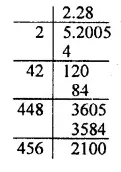Required square root = 2.28 upto two places of decimal.

(vi) 0.602Required square root = 0.78 upto two places of decimals.

4. Find the square root of each of the following correct to two decimal places:
(i)$3\frac { 4 }{ 5 }$
(ii)$6\frac { 7 }{ 8 }$
Solution
(i) 3 4/5 = 3.80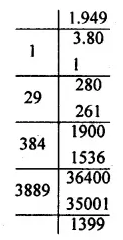Reqd. square root = 1.949 = 1.95 upto two places of decimal

(ii) 6 7/8 = 6.875Reqd. square root = 2.62

5. For each of the following, find the least number that must be subtracted so that the resulting number is a perfect square.
(i) 796
(ii) 1886
(iii) 23497
Solution
(i) 796
Taking square root of 796, we find that 12 has been left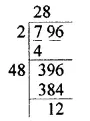∴ Least number to be subtracted = 12

(ii) 1886
Taking square root of 1886, we find that 37 has been left∴ Least number to be subtracted = 37

(iii) 23497
Taking square root of 23497, we find that 88 has been left∴ Least number to be subtracted = 88

6. For each of the following, find the least number that must be added so that the resulting number is a perfect square.
(i) 511
(ii) 7172
(iii) 55078
Solution
(i) 511
Taking square root of 511, we find that 27 has been left
We see that 511 is greater than (22)2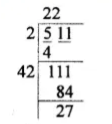On adding the required number to 511, we get (23)2 i.e., 529
So, the required number = 529 – 511 = 18

(ii) 7172
Taking square root of 7172, we find that 116 has been left
We see that 7172 is greater than (84)2∴ On adding the required number to 7172, we get (85)2 i.e., 7725
Required number = 7225 – 7172 = 53

(iii) 55078Taking square root of 55078, we find that 322 has been lest
We see that 55078 is greater than (234)2
On adding the required number to 55078, we get (235)2  i.e., 55225
Required number = 55225 – 55078 = 147

7. Find the square root of 7 correct to two decimal places; then use it to find the value ofcorrect to three significant digits.
Solution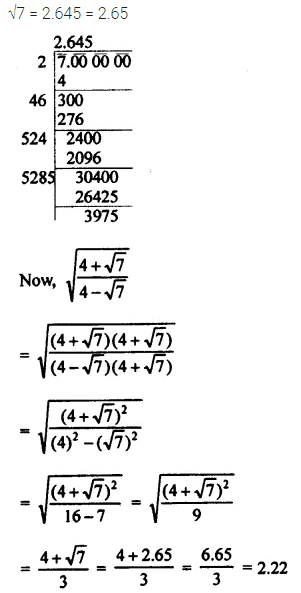8. Find the value of √5 correct to 2 decimal places; then use it to find the square root of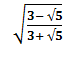correct to 2 significant digits.
Solution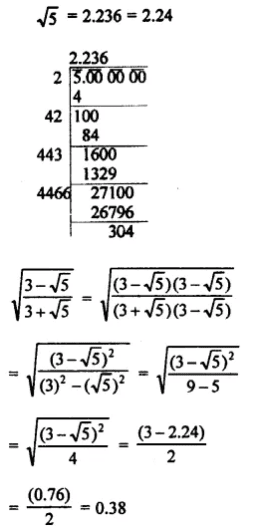9. Find the square root of:
(i) 1764/2809
(ii) 507/4107
(iii)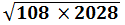(iv) 0.01 + √0.0064
Solution
(i)(ii)(iii)(iv)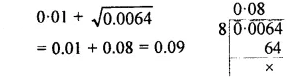10. Find the square root of 7.832 correct to :
(i) 2 decimal places
(ii) 2 significant digits.
Solution
Square root of 7.832√7.832 = 2.80 upto two decimal places = 2.8 upto two significant places.

11. Find the least number which must be subtracted from 1205 so that the resulting number is a perfect square.
Solution
Clearly, if 49 is subtracted from 1205, the number will be a perfect square.∴ 1205 – 49 = 1156 and √(1156 ) = 34

12. Find the least number which must be added to 1205 so that the resulting number is a perfect square.
Solution
Clearly, 1205 is greater than 342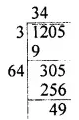∴ On adding the required number to 1205, we shall be getting 352 i.e., 1225
∴ The  required number = 1225 – 1205 = 20

13. Find the least number which must be subtracted from 2037 so that the resulting number is a perfect square.
Solution
Clearly; if 12 is subtracted from 2037, the remainder will be a perfect square.
∴ 2037 – 12 = 2035 and √2035 = 4514. Find the least number which must be added to 5483 so that the resulting number is a perfect square.
Solution
Clearly, 5483 is greater than 742.
∴ On adding the required number to 5483, we shall be getting 752 i.e., 5625.
Hence, the required number = 5625 – 5483
= 142

### Exercise 3 C

1. Seeing the value of the digit at unit’s place, state which of the following can be square of a number :
(i) 3051
(ii) 2332
(iii) 5684
(iv) 6908
(v) 50699
Solution
We know that the ending digit (the digit at units place) of the square of a number is 0, 1, 4, 5, 6 or 9
So, the following numbers can be squares.
3051, 5684 and 50699 i.e., (i), (iii), and (v)

2. Squares of which of the following numbers will have 1 (one) at their unit’s place:
(i) 57
(ii) 81
(iii) 139
(iv) 73
(v) 64
Solution
The square of the following numbers will have 1 at their units place as
(1)2 = 1, (9)2 = 81
81 and 139 i.e., (i) and (iii)

3. Which of the following numbers will not have 1 (one) at their unit’s place:
(i) 322
(ii) 572
(iii) 692
(iv) 3212
(v) 2652
Solution
The square of the following numbers will not have 1 at their units place:
As only (1)2 = 1, (9)2 = 81 have at then units place
322, 572, 2652 i.e., (i), (ii) and (v)

4. Square of  which of the following numbers will not have 6 at their unit’s place:
(i) 35
(ii) 23
(iii) 64
(iv) 76
(v) 98
Solution
The squares of the following  numbers,
Will not have 6 at their units place as only (4)2 = 16, (6)2 = 36 has but its units place 35, 23 and 98 i.e., (i), (ii), and (v)

5. Which of the following numbers will have 6 at their unit’s place:
(i) 262
(ii) 492
(iii) 342
(iv) 432
(v) 2442
Solution
The following numbers have 6 at their units place as (4)2 = 16, (6)2 = 36 has 6 at their units place 262, 342, 2442 i.e., (i), (ii) and (v)

6. If a number ends with 3  zeroes, how many zeroes will its square have?
Solution
We know that if a number ends with n zeroes, then its square will have 2n zeroes at their ends
A number ends with 3 zeroes, then its square will have 3 × 2 = 6 zeroes.

7. If the square of a number ends with 10 zeroes, how many zeroes will the number have?
Solution
We know that if a number ends with n zeroes
Then its square will have 2n zeroes Conversely.
If square of a number have 2n zeroes at their ends then the number will have n zeroes
The square of a number ends 10 zeroes,
Then the number will have 10/2 = 5 zeroes

8. Is it possible for the square of a number to end with 5 zeroes ? Give reason.
Solution
No, it is not possible for the square of a number, to have  5 zeroes which is odd because the number of zeroes of the square must be 2n zeroes i.e., even number of zeroes.

9. Give reason to show that none of the numbers, given below, is a perfect square.
Solution
A number having 2, 3, 7 or 8 at the unit place is never a perfect square.

10. State, whether the square of the following numbers is even or odd?
(i) 23
(ii) 54
(iii) 76
(iv) 75
Solution
(i) 23 - odd
(ii) 54 - even
(iii) 76 - odd
(iv) 75 - even

11. Give reason to show that none of the numbers 640, 81000 and 3600000 is a perfect square.
Solution
No, number has an even number of zeroes.

12. Evaluate:
(i) 372 - 362
(ii) 852 – 842
(iii) 1012 – 1002
Solution
(i) 372 – 362
Using property, for any natural number n,
(n + 1)2 – n2 = (n + 1) + n
⇒ (36 + 1)2 – 362 = (36 + 1) + 36
⇒  372 – 362 = 37 + 36
⇒  372 – 362 = 73

(ii) 852 – 842
Using property, for any natural number n,
(n + 1)2 – n2 = (n + 1) + n
⇒ (84 + 1)2 – 842 = (84 + 1) + 84
⇒ 852 – 842 = 85 + 84
⇒ 852 – 842 = 169

(iii) 1012 – 1002
Using property, for any natural number n,
(n + 1)2 – n2 = (n + 1) + n
⇒ (100 + 1)2 – 1002 = (100 + 1) + 100
⇒ 1012 – 1002 = 101 + 100
⇒ 1012 – 1002 = 201

13. Without doing the actual addition, find the sum of:
(i) 1 + 3 + 5 + 7 + 9 + 11 + 13 + 15 + 17 + 19 + 21 + 23
(ii) 1 + 3 + 5 + 7 + 9 + ....... + 39 + 41
(iii) 1 + 3 + 5 + 7 + 9 + ....... + 51 + 53
Solution
(i) 1 + 3 + 5 + 7 + 9 + 11 + 13 + 15 + 17 + 19 + 21 + 23 = Sum of first 12 odd natural numbers = 122 = 144
(ii) 1 + 3 + 5 + 7 + 9 + …… + 39 + 41 = sum of first 21 odd numbers = 212 = 441
(iii) 1 + 3 + 5 + 7 + 9 + …. + 51 + 53  = sum of  first 27 odd natural number = 272 = 729

14. Write three sets of Pythagorean triplets such that each set has numbers less than 30.
Solution
The three sets of Pythagorean triplets such that each set has numbers less than 30 are 3, 4 and 5, 6, 8 and 10; 5, 12 and 13
Proof :
In 3, 4 and 5
32 + 42 = 52
⇒ 9 + 16 = 25
⇒ 25 = 25
In 6, 8 and 10
62 + 82 = 102
⇒ 36 + 64 = 100
⇒ 100 = 100
In 5, 12 and 13
52 + 122 = 132
⇒ 25 + 144 = 169
⇒ 169 = 169